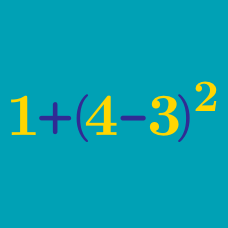Everyday Math

# Multiplication and Division

What is the value of $7 \times 8$?

What is the value of $42 \div 7$?

What is the value of $9 \times 0$?

What is the value of $720 \div 8$?

What is the value of $21 \times 2$?

×